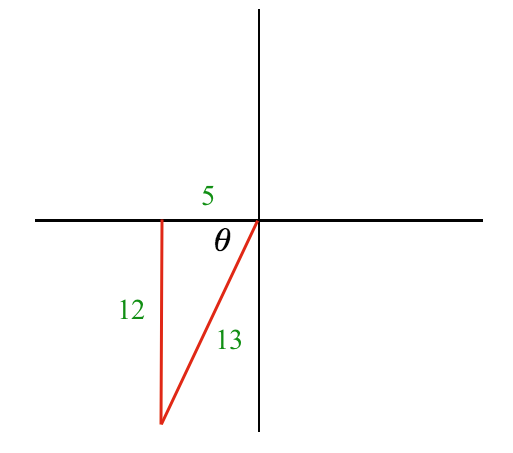### Home > INT3 > Chapter 10 > Lesson 10.3.3 > Problem10-189

10-189.

Given the points $O\left(0, 0\right)$ and $R\left(–5, –12\right)$:

1. What is the equation of the circle centered at point $O$ and passing through point $R$?

Determine the radius of the circle.
$r = 13$

Use the general equation of a circle:
$\left(x − h\right)^{2} + \left(y − k\right)^{2} = r^{2}$

2. What is the measure of the angle formed by $\overline { O R }$ and the positive $x$-axis? Answer in both radians and degrees.

Use a trigonometric function to determine the angle $θ$.3. Graph your circle in part (a) and $x^{2} + y^{2} = 144$ on the same set of axes. Shade the region between the circles.

Create a graph.

4. If we use these circles as a dart board and the dart is certain to hit randomly somewhere within the largest circle, what is the probability of hitting the shaded region?

Determine the area of the shaded area and the total area.